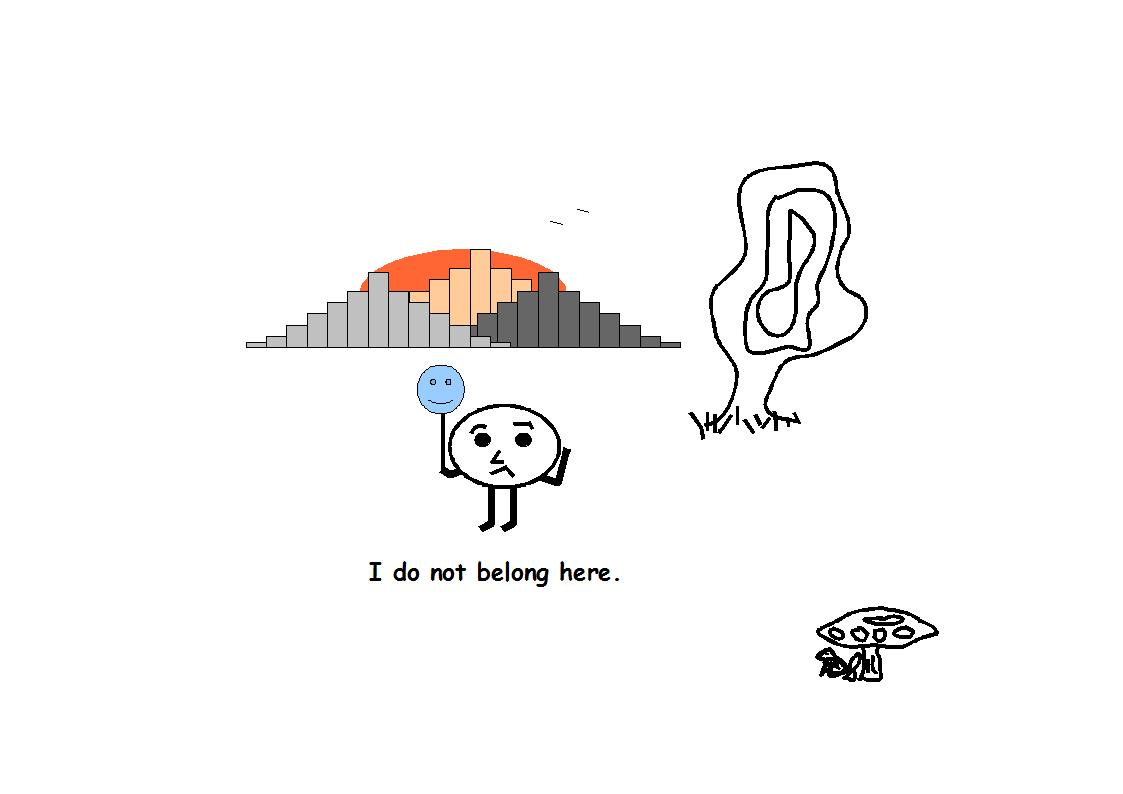# ijspace.org

 In a Nutshell-2014   28th December 2014       As the year 2014 is winding down, we provide a summary of the information presented on www.ijspace.org. We recall that this exercise in futility started with rather innocuous query regarding the slowest continuously moving object in nature known to us. As a consequence we had to envision a space where the objects were described on the basis of measurements. Each and every entity (microscopic and macroscopic, singular and ensemble) in this description was in a state of measurement. An entity was an observer and an object both, making measurements and being measured simultaneously.     We immediately ran into the problem of the definition of the origin which obviously cannot be measured accurately. So the measurements were described based on the observer's capacity to measure them. This led to a rather interesting concept of Virtual Twin Symmetry (VTS), which allowed us to create the lower limit on the physical dimensions and an upper limit on the capacity of each observer (At some point we will discuss this duality in detail, however it will suffice to say that something similar is probably being said in string theory as U-symmetry.)     We were able to describe a discrete measurement space (j-space), for a finite capacity observer. We had our own version of Maxwell's demon with capacity to perform zero-entropy measurement in the form of Obsi. One of the outcome was our ability to understand the significance of the fine-structure constant alpha phenomenologically without having to resort to the physical constants such as e, h, and c. More importantly it appeared that the value of alpha corresponded to what we could call q=3 state. So we could only imagine what would be our description of the universe for q=2 state which was a higher information state.     An interesting result was the evaluation of the angle ΘEH  between E and H vectors, which had to be either greater than or less than 90o in j-space. The angle ΘEH  was likely to be greater than 90o. This allowed us some insight into the 3-D constraints of the QED universe we existed in.     The fundamental problem had been the definitions of time and continuity. The time issue was resolved by moving away from concept of time as a universal entity. We assigned time to each observer as part of the universe the observer was able to measure. We considered the universe as we knew it as a single measurement itself if measured by Obsi. Each observer had its own measurement metric and corresponding (t, x, y, z, m) parameters. As a consequence the entropy of the measurement can be correlated to the time. More difficult the measurement, higher the entropy and therefore higher the length of time axis. Any macroscopic observer would not be able to complete the measurements due to inherent entropy and hence the time axis could be considered infinite. In j-space the time could not exist without a measurement or entropy. The problem of continuity is not as straightforward and it is still being worked upon.     The force fields in j-space will never be irrotational and divergence free, i.e. the properties like angular momentum and mass (source) will be intrinsic to the description. Also symmetries represent the limitation of the measurements for an observer. The most fundamental symmetry will be the symmetry defining the origin which is the VTS symmetry. Another important aspect is the calculation of the integrals and their limits. The limits are always finite. For different phenomena the limits can be replaced by the values which are experimentally determined.     We have discussed some of the issues which are of interest to us. We still have many aspects of nature which we do not understand. i. How accurate is our assumption of ∫|Ψ |2 dX = 1, in describing a phenomenon in a space with entropy? Can we really describe the quantum entanglement using Ψ ? ii. Can we understand the concept of z-axis better? iii. What  do the constants such as Planck's and Boltzmann's really represent in an information space with entropy? Why is the speed of light finite? Is it because of Observer's capacity or is it the way the dice has been rolled? iv. Can we develop a phenomenological description independent of (t, x, y, z, m)? v. The infinite fulcrum may describe characteristics of Andromeda galaxy, but are there structures at microscopic level which follow the similar characteristics? vi. What does continuity really mean? Under what conditions can we draw a true straight line or a circle, and develop the concept of convergence? This should lead to the development of geometrical structures.     Finally the anhilation-creation operators of Quantum Mechanics or Kant's distinction between noumenon and phenomenon? Kant definitely has an edge there. Let us wish for a much better 2015 than 2014 has been, especially for children.